# Self Organizing Maps

Tom Germano
March 23, 1999

### Table Of Conents

I. Introduction to Self-Organizing Maps
II. Components
A. Sample Data
B. Weights
III. Main Algorithm
A. Initializing the Weights
B. Get Best Matching Unit
C. Scale Neighbors
1. Determining Neighbors
2. Learning
IV. Determining the Quality of SOMs
V. Java Example
VI. Conclusions
VII. References
A. Books
B. Papers

### I. Introduction

Self-organizing maps (SOMs) are a data visualization technique invented by Professor Teuvo Kohonen which reduce the dimensions of data through the use of self-organizing neural networks. The problem that data visualization attempts to solve  is that humans simply cannot visualize high dimensional data as is so techniques are created to help us understand this high dimensional data. Two other techniques of reducing the dimensions of data that has been presented in this course has been N-Land and Multi-dimensional Scaling. The way  SOMs go about reducing dimensions is by producing a map of usually 1 or 2 dimensions which plot the similarities of the data by grouping similar data items together.  So SOMs accomplish two things, they reduce dimensions and display similarities.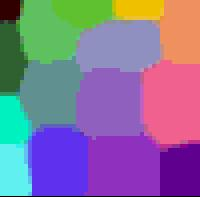Just to give you an idea of what a SOM looks like, here is an example of a SOM which I constructed.  As you can see, like colors are grouped together such as the greens are all in the upper left hand corner and the purples are all grouped around the lower right and right hand side.

### A. Sample Data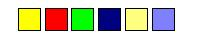The first part of a SOM is the data. Above are some examples of 3 dimensional data which are commonly used when experimenting with SOMs. Here the colors are represented in three dimensions (red, blue, and green.) The idea of the self-organizing maps is to project the n-dimensional data (here it would be colors and would be 3 dimensions) into something that be better understood visually (in this case it would be a 2 dimensional image map). In this case one would expect the dark blue and the greys to end up near each other on a good map and yellow close to both the red and the green.

### B. Weights

The second component to SOMs are the weight vectors. Each weight vector has two components to them which I have here attempted to show in the image below.  The first part of a weight vector is its data. This is of the same dimensions as the sample vectors and the second part of a weight vector is its natural location. The good thing about colors is that the data can be shown by displaying the color, so in this case the color is the data, and the location is the x,y position of the pixel on the screen.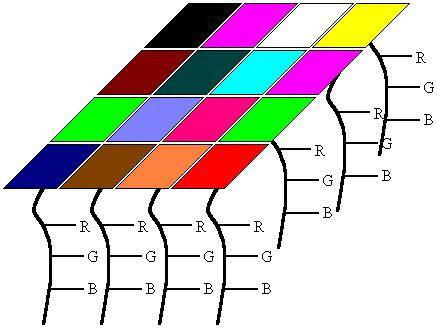In this example, I tried to show what a 2D array of weight vectors would look like. This picture is a skewed view of a grid where you have the n-dimensional array for each weight and each weight has its own unique location in the grid. Weight vectors dont necessarily have to be arranged in 2 dimensions, a lot of work has been done using SOMs of 1 dimension, but the data part of the weight must be of the same dimensions as the sample vectors.Weights are sometimes referred to as neurons since SOMs are actually neural networks.

### III. Main Algorithm

The way that SOMs go about organizing themselves is by competeting for representation of the samples. Neurons are also allowed to change themselves by learning to become more like samples in hopes of winning the next competition. It is this selection and learning process that makes the weights organize themselves into a map representing similarities.

So with these two components (the sample and weight vectors), how can one order the weight vectors in such a way that they will represent the similarities of the sample vectors? This is accomplished by using  the very simple algorithm shown here.

Initialize Map
For t from 0 to 1
Randomly select a sample
Get best matching unit
Scale neighbors
Increase t a small amount
End for

The first step in constructing a SOM  is to initialize the weight vectors. From there you select a sample vector randomly and search the map of weight vectors to find which weight best represents that sample. Since each weight vector has a location, it also has neighboring weights that are close to it. The weight that is chosen is rewarded by being able to become more like that randomly selected sample vector. In addition to this reward, the neighbors of that weight are also rewarded by being able to become more like the chosen sample vector. From this step we increase t some small amount because the number of neighbors and how much each weight can learn decreases over time. This whole process is then repeated a large number of times, usually more than 1000 times.

In the case of colors, the program would first select a color from the array of samples such as green, then search the weights for the location containing the greenest color. From there, the colors surrounding that weight are then made more green. Then another color is chosen, such as red, and the process continues.

### A. Initializing the Weights

Here are screen shots of the three different ways which I decided to initialize the weight vector map. I should first mention the palette here. In the java program below there are 6 intensities of red, blue, and green displayed, it really doesnt take away from the visual experience. The actual values for the weights are floats, so they have a bigger range than the six values that are shown.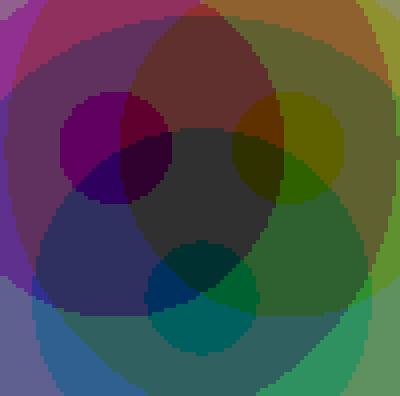There are a number of ways to initialize the weight vectors. The first you can see is just give each weight vector random values for its data. A screen of pixels with random red, blue, and green values are shown above on the left. Unfortunately calculating SOMs is very computationally expensive, so there are some variants of initializing the weights so that samples that you know for a fact are not similar start off far away. This way you need less iterations to produce a good map and can save yourself some time.

Here I made two other ways to initialize the weights in addition to the random one. This one is just putting red, blue, green, and black at all four corners and having them slowly fade toward the center.  This other one is having red, green, and blue equally distant from one another and from the center.

### B. Get Best Matching Unit

This is a very simple step, just go through all the weight vectors and calculate the distance from each weight to the chosen sample vector. The weight with the shortest distance is the winner. If there are more than one with the same distance, then the winning weight is chosen randomly among the weights with the shortest distance.  There are a number of different ways for determining what distance actually means mathematically.  The most common method is to use the Euclidean distance:where x[i] is the data value at the ith data member of a sample and n is the number of dimensions to the sample vectors.

In the case of colors, if we can think of them as 3D points, each component being an axis. If we have chosen green which is of the value (0,6,0), the color light green (3,6,3) will be closer to green than red at (6,0,0).

Light green = Sqrt((3-0)^2+(6-6)^2+(3-0)^2) = 4.24
Red           = Sqrt((6-0)^2+(0-6)^2+(0-0)^2) =  8.49

So light green is now the best matching unit, but this operation of calculating distances and comparing them is done over the entire map and the wieght with the shortest distance to the sample vector is the winner and the BMU. The square root is not computed in the java program for speed optimization for this section.

### 1. Determining Neighbors

There are actually two parts to scaling the neighboring weights: determining which weights are considered as neighbors and how much each weight can become more like the sample vector. The neighbors of a winning weight can be determined using a number of different methods. Some use concentric squares, others hexagons, I opted to use a gaussian function where every point with a value above zero is considered a neighbor.

As mentioned previously, the amount of neighbors decreases over time. This is done so samples can first move to an area where they will probably be, then they jockey for position. This process is similar to coarse adjustment followed by fine tuning. The function used to decrease the radius of influence doesnt really matter as long as it decreases, I just used a linear function.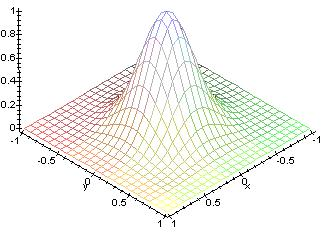This picture shows a plot of the function used. As the time progresses, the base goes towards the center, so there are less neighbors as time progresses. The initial radius is set really high, some value near the width or height of the map.

### 2. Learning

The second part to scaling the neighbors is the learning function. The winning weight is rewarded with becoming more like the sample vector.  The neighbors also become more like the sample vector. An attribute of this learning process is that the farther away the neighbor is from the winning vector, the less it learns. The rate at which the amount a weight can learn decreases and can also be set to whatever you want. I chose to use a gaussian function. This function will return a value ranging between 0 and 1, where each neighbor is then changed using the parametric equation.  The new color is:

Current color*(1.-t) + sample vector*t

So in the first iteration, the best matching unit will get a t of 1 for its learning function, so the weight will then come out of this process with the same exact values as the randomly selected sample.

Just as the amount of neighbors a weight has falls off, the amount a weight can learn also decreases with time. On the first iteration, the winning weight becomes the sample vector since t has a full range of from 0 to 1. Then as time progresses, the winning weight becomes slightly more like the sample where the maximum value of t decreases. The rate at which the amount a weight can learn falls of linearly. To depict this visually, in the previous plot, the amount a weight can learn is equivalent to how high the bump is at their location.  As time progresses, the height of the bump will decrease. Adding this function to the neighborhood function will result in the height of the bump going down while the base of the bump shrinks.

So once a weight is determined the winner, the neighbors of that weight are found and each of those neighbors in addition to the winning weight change to become more like the sample vector.

### IV. Determining the Quality of SOMsAbove is another example of a SOM generated by the java program using 500 iterations. At first glance you will notice that similar colors are all grouped together yet again. However, this is not always the case as you can see that there are some colors who are surrounded by colors that are nothing like them at all. It may be easy to point this out with colors since this is something that we are familiar with, but if we were using more abstract data, how would we know that since two entities are close to each other means that they are similar and not that they are just there because of bad luck?

There is a very simple method for displaying where similarities lie and where they do not. In order to compute this we go through all the weights and determine how similar the neighbors are. This is done by calculating the distance that the weight vectors make between the each weight and each of its neighbors. With an average of these distances a color is then assigned to that location. This procedure is located in Screen.java and named public void update_bw().

If the average distance were high, then the surrounding weights are very different and a dark color is assigned to the location of the weight. If the average distance is low, a lighter color is assigned. So in areas of the center of the blobs the colors are the same, so it should be white since all the neighbors are the same color. In areas between blobs where there are similarities it should be not white, but a light grey. Areas where the blobs are physically close to each other, but are not similar at all there should be black.As shown above, the ravines of black show where the colors may be physically close to each other on the map, but are very different from each other when it comes to the actual values of the weights. Areas where there is a light grey between the blobs represent a true similarity.  In the pictures above, in the bottom right there is black surrounded by colors which are not very similar to it. When looking at the black and white similarity SOM, it shows that black is not similar to the other colors because there are lines of black representing no similarity between those two colors. Also in the top corner there is pink and nearby is a light green which are not very near each other in reality, but near each other on the colored SOM. Looking at the black and white SOM, it clearly shows that the two not very similar by having black in between the two colors.

With these average distances used to make the black and white map, we can actually assign each SOM a value that determines how good the image represents the similarities of the samples  by simply adding these averages.

### V. Java Example

In order to help others understand how SOMs look when organizing themselves and how they look when they finally settle, I coded up this java applet called Self-Organizing Maps. This program was programmed for Suns JDK 1.2, so in order to view it you will need to install the Java Runtime Environment 1.2 .

The source is made up of the following three files:

soms.java
Screen.java
fpoint.java
The sources and HTML files are all bundled into this file: wpi-soms.zip

### VI. Conclusions

Probably the best thing about SOMs that they are very easy to understand. Its very simple, if they are close together and there is grey connecting them, then they are similar. If there is a black ravine between them, then they are different. Unlike Multidimensional Scaling or N-land, people can quickly pick up on how to use them in an effective manner.

Another great thing is that they work very well. As I have shown you they classify data well and then are easily evaluate for their own quality so you can actually calculated how good a map is and how strong the similarities between objects are.

One major problem with SOMs is getting the right data. Unfortunately you need a value for each dimension of each member of samples in order to generate a map. Sometimes this simply is not possible and often it is very difficult to acquire all of this data so this is a limiting feature to the use of SOMs often referred to as missing data.

Another problem is that every SOM is different and finds different similarites among the sample vectors. SOMs organize sample data so that in the final product, the samples are usually surrounded by simliar samples, however similar samples are not always near each other. If you have a lot of shades of purple, not always will you get one big group with all the purples in that cluster, sometimes the clusters will get split and there will be two groups of purple. Using colors we could tell that those two groups in reality are similar and that they just got split, but with most data, those two clusters will look totally unrelated. So a lot of maps need to be constructed in order to get one final good map.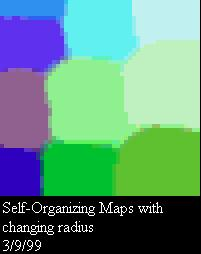Heres an example of just this sort of exact problem happening.  You can see in the picture on the left that there are three shades of purple that are all in one column. Yes those three colors are very similar, but two deep shades of purple are split by a lighter shade of purple. The deep purples are more similar to each other and should therefor be near one another as shown in the picture on the right.

The final major problem with SOMs is that they are very computationally expensive which is a major drawback since as the dimensions of the data increases, dimension reduction visualization techniques become more important, but unfortunately then time to compute them also increases. For calcuating that black and white similarity map, the more neighbors you use to calculate the distance the better similarity map you will get, but the number of distances the algorithm needs to compute increases exponentially.

### A. Books